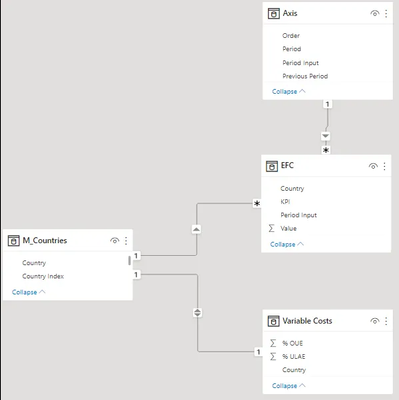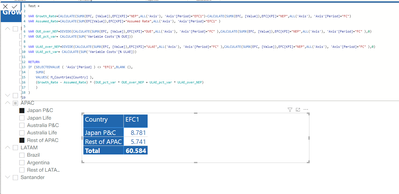cancel
Showing results for
Did you mean:Helper I

## Roll-Up total doesn't add up

Hi,

I'm having a hard time figuring out why my total doesn't add up. Would anyone be able to help me figure out how to fix it ?
The DAX for the total probably should have a different expression, hence the switch(), but I just don't know what would be correct.Thanks!

1 ACCEPTED SOLUTIONSuper User

``````Test =
SUMX(
VALUES( M_Countries[Country] ),

VAR Growth_Rate =
CALCULATE(SUM(EFC[Value]),EFC[KPI]="NEP",ALL('Axis'), 'Axis'[Period]="EFC1")
- CALCULATE(SUM(EFC[Value]),EFC[KPI]="NEP",ALL('Axis'), 'Axis'[Period]="FC")

VAR Assumed_Rate=
CALCULATE(SUM(EFC[Value]),EFC[KPI]="Assumed Rate", ALL('Axis'), 'Axis'[Period]="EFC1" )

VAR OUE_over_NEP =
DIVIDE(
CALCULATE(SUM(EFC[Value]),EFC[KPI]="OUE",ALL('Axis'), 'Axis'[Period]="FC" ),
CALCULATE(SUM(EFC[Value]),EFC[KPI]="NEP",ALL('Axis'), 'Axis'[Period]="FC" )
,0)

VAR OUE_pct_var =
CALCULATE(SUM('Variable Costs'[% OUE]))

VAR ULAE_over_NEP=
DIVIDE(
CALCULATE(SUM(EFC[Value]),EFC[KPI]="ULAE",ALL('Axis'), 'Axis'[Period]="FC" ),
CALCULATE(SUM(EFC[Value]),EFC[KPI]="NEP",ALL('Axis'), 'Axis'[Period]="FC" )
,0)

VAR ULAE_pct_var= CALCULATE(SUM('Variable Costs'[% ULAE]))

RETURN

IF (
SELECTEDVALUE ( 'Axis'[Period] ) <> "EFC1",
BLANK (),
(Growth_Rate - Assumed_Rate) * (OUE_pct_var * OUE_over_NEP + ULAE_pct_var * ULAE_over_NEP)
)
)``````

You'd closed the SUMX to early. Hope the above now works!

Ben Dobbs

Did I answer your question? Mark my post as a solution! This will help others on the forum!
10 REPLIES 10Helper ISuper User

Ignore request for PBIX File.

Try this:

``````SUMX(
VALUES( M_Countries[Country] ),

VAR = ....
VAR = ....
VAR = ....

RETURN (Growth_Rate - Assumed_Rate) * (OUE_pct_var * OUE_over_NEP + ULAE_pct_var * ULAE_over_NEP)
)``````

The VAR = bits is you existing code. I've just wrapped the whole thing in an iterator over countries.

Ben Dobbs

Did I answer your question? Mark my post as a solution! This will help others on the forum!Helper II hope I understood you well. If so, it still does not work.
I can t find a way to share the Power BI, the company is pretty strict. I'm waiting for an approval to share dummy data though.Super User

Not quite, you needed a single SUMX round the whole lot. If you paste just the DAX code in text form I'll edit and send back.

Ben Dobbs

Did I answer your question? Mark my post as a solution! This will help others on the forum!Helper I

Hmm, I did try at first this way but DAX errorHere's the text:

``````Test =
SUMX(VALUES( M_Countries[Country] ),
VAR Growth_Rate=CALCULATE(SUM(EFC[Value]),EFC[KPI]="NEP",ALL('Axis'), 'Axis'[Period]="EFC1")
- CALCULATE(SUM(EFC[Value]),EFC[KPI]="NEP",ALL('Axis'), 'Axis'[Period]="FC")
VAR Assumed_Rate=CALCULATE(SUM(EFC[Value]),EFC[KPI]="Assumed Rate",ALL('Axis'), 'Axis'[Period]="EFC1" )

VAR OUE_over_NEP= DIVIDE(CALCULATE(SUM(EFC[Value]),EFC[KPI]="OUE",ALL('Axis'), 'Axis'[Period]="FC" ),CALCULATE(SUM(EFC[Value]),EFC[KPI]="NEP",ALL('Axis'), 'Axis'[Period]="FC" ),0)
VAR OUE_pct_var= CALCULATE(SUM('Variable Costs'[% OUE]))

VAR ULAE_over_NEP=DIVIDE(CALCULATE(SUM(EFC[Value]),EFC[KPI]="ULAE",ALL('Axis'), 'Axis'[Period]="FC" ),CALCULATE(SUM(EFC[Value]),EFC[KPI]="NEP",ALL('Axis'), 'Axis'[Period]="FC" ),0)
VAR ULAE_pct_var= CALCULATE(SUM('Variable Costs'[% ULAE]))
)
RETURN
IF (SELECTEDVALUE ( 'Axis'[Period] ) <> "EFC1",BLANK (),
(Growth_Rate - Assumed_Rate) * (OUE_pct_var * OUE_over_NEP + ULAE_pct_var * ULAE_over_NEP))``````Super User

``````Test =
SUMX(
VALUES( M_Countries[Country] ),

VAR Growth_Rate =
CALCULATE(SUM(EFC[Value]),EFC[KPI]="NEP",ALL('Axis'), 'Axis'[Period]="EFC1")
- CALCULATE(SUM(EFC[Value]),EFC[KPI]="NEP",ALL('Axis'), 'Axis'[Period]="FC")

VAR Assumed_Rate=
CALCULATE(SUM(EFC[Value]),EFC[KPI]="Assumed Rate", ALL('Axis'), 'Axis'[Period]="EFC1" )

VAR OUE_over_NEP =
DIVIDE(
CALCULATE(SUM(EFC[Value]),EFC[KPI]="OUE",ALL('Axis'), 'Axis'[Period]="FC" ),
CALCULATE(SUM(EFC[Value]),EFC[KPI]="NEP",ALL('Axis'), 'Axis'[Period]="FC" )
,0)

VAR OUE_pct_var =
CALCULATE(SUM('Variable Costs'[% OUE]))

VAR ULAE_over_NEP=
DIVIDE(
CALCULATE(SUM(EFC[Value]),EFC[KPI]="ULAE",ALL('Axis'), 'Axis'[Period]="FC" ),
CALCULATE(SUM(EFC[Value]),EFC[KPI]="NEP",ALL('Axis'), 'Axis'[Period]="FC" )
,0)

VAR ULAE_pct_var= CALCULATE(SUM('Variable Costs'[% ULAE]))

RETURN

IF (
SELECTEDVALUE ( 'Axis'[Period] ) <> "EFC1",
BLANK (),
(Growth_Rate - Assumed_Rate) * (OUE_pct_var * OUE_over_NEP + ULAE_pct_var * ULAE_over_NEP)
)
)``````

You'd closed the SUMX to early. Hope the above now works!

Ben Dobbs

Did I answer your question? Mark my post as a solution! This will help others on the forum!Helper I

THANK YOU ! 😍Super User

Are you able to supply a pbix file with some dummy data in it?

Ben Dobbs

Did I answer your question? Mark my post as a solution! This will help others on the forum!Super User

Replace the return with this:

``````RETURN

SUMX(
M_Countries[Country],
(Growth_Rate - Assumed_Rate) * (OUE_pct_var * OUE_over_NEP + ULAE_pct_var * ULAE_over_NEP)
)
``````

It forces the calculation to be done one country at the time and then adds the result.

Within a row there is only one country so you get the result back. In the total it adds up each country.

Ben Dobbs

Did I answer your question? Mark my post as a solution! This will help others on the forum!Super User

Sorry I missed a VALUES!

``````RETURN

SUMX(
VALUES( M_Countries[Country] ),
(Growth_Rate - Assumed_Rate) * (OUE_pct_var * OUE_over_NEP + ULAE_pct_var * ULAE_over_NEP)
)``````

Ben Dobbs

Did I answer your question? Mark my post as a solution! This will help others on the forum!Announcements#### Exclusive opportunity for Women!

Join us for a free, hands-on Microsoft workshop led by women trainers for women where you will learn how to build a Dashboard in a Day!#### Power Platform Conference-Power BI and Fabric Sessions

Join us Oct 1 - 6 in Las Vegas for the Microsoft Power Platform Conference.Top Solution Authors
Top Kudoed Authors
Users online (1,729)